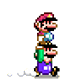## 初期准备

• 海康威视网络摄像机一台（基于海康SDK开发）
• 电脑一台（废话）
• 叫花鸡（jiāo huàn jī，手抖打错，感觉挺有意思就不改了，非必须）
• 支持S7协议的PLC一台（做这个东西的本意就是报警输出至PLC，否则用自带软件就可以了）
• C#开发环境
• .NET Framework 4.5.2以上（本文4.7.2）
• 海康SDK（官网提供下载

## 处理图片

### 抓取图片

wPicQuality 图片质量系数：0-最好，1-较好，2-一般
wPicSize 图片尺寸：5-HD720P(1280*720)，9-HD1080P(1920*1080)，0xff-Auto(使用当前码流分辨率)

private Image JpgtoBuffer()
{
byte[] jpgBytes = new byte;//1920*1080的jpg图像大小约400000字节
UInt32 sizeReturned = 0;
int lChannel = 1; //通道号
CHCNetSDK.NET_DVR_JPEGPARA lpJpegPara = new CHCNetSDK.NET_DVR_JPEGPARA
{
wPicQuality = 0, //图像质量
wPicSize = 0xff //抓图分辨率
};
if (!CHCNetSDK.NET_DVR_CaptureJPEGPicture_NEW(handle,lChannel,ref lpJpegPara,jpgBytes, (uint)jpgBytes.Length,ref sizeReturned))
{
iLastErr = CHCNetSDK.NET_DVR_GetLastError();
str = "失败，错误代码：" + iLastErr;
MessageBox.Show(str, "提示");
return null;
}
byte[] realBytes = new byte[sizeReturned];
Buffer.BlockCopy(jpgBytes, 0, realBytes, 0, realBytes.Length);
MemoryStream ms = new MemoryStream(realBytes);
Image pic = Image.FromStream(ms);
return pic;
}

### 缩小尺寸

private Image smallPic(Image bigPic)
{
Image newsmall = bigPic.GetThumbnailImage(32, 32, () => { return false; }, IntPtr.Zero);
return newsmall;
}

### 计算灰度

private Byte[] ToGray(Image input)
{
Bitmap bit = new Bitmap(input);
Byte[] gray = new Byte[input.Width * input.Height];
for (int i = 0; i < input.Width; i++)
{
for (int j = 0; j < input.Height; j++)
{
Color color = bit.GetPixel(i, j);
byte grayvalue = (Byte)(color.R * 0.3 + color.G * 0.59 + color.B * 0.11);
gray[i * input.Width + j] = grayvalue;
}
}
return gray;
}

private Bitmap GrayScale(Bitmap original)
{
Bitmap newBitmap = new Bitmap(original.Width, original.Height);
Graphics g = Graphics.FromImage(newBitmap);
ColorMatrix colorMatrix = new ColorMatrix(
new float[][]
{
new float[]{.30f,.30f,.30f,0,0 },
new float[]{.59f,.59f,.59f,0,0 },
new float[]{.11f,.11f,.11f,0,0 },
new float[]{0,0,0,1,0 },
new float[]{0,0,0,0,1 }
});
ImageAttributes attributes = new ImageAttributes();
attributes.SetColorMatrix(colorMatrix);
g.DrawImage(original, new Rectangle(0, 0, original.Width, original.Height), 0, 0, original.Width, original.Height, GraphicsUnit.Pixel, attributes);
g.Dispose();
return newBitmap;
}

### 计算指纹

private Byte GrayAvg(Byte[] values)
{
int sum = 0;
for(int i = 0; i < values.Length; i++)
{
sum += (int)values[i];
}
return Convert.ToByte(sum / values.Length);
}

private string GrayHash(byte[] value, byte avg)
{
char[] result = new char[value.Length];
for(int i = 0; i < value.Length; i++)
{
if (value[i] > avg)
{
result[i] = '1';
}
else
{
result[i] = '0';
}
}
string hash = new string(result);
return hash;
}

private Int32 CountSameNum(string last, string now)
{
int count = 0;
if (last.Length != now.Length)
{
StatusLabel.Text = "运算错误";
}
else
{
for (int i = 0; i < last.Length; i++)
{
if (last[i] != now[i])
{
count++;
}
}
}
return count;
}

## 输出信号

private string lastHash;//上一张图的指纹private string nowHash;//最新一张图的指纹private Image nowImg;//当前图像private Plc plc;//PLC

lastHash = nowHash;
nowImg = JpgtoBuffer();
Image smallOne = smallPic(nowImg);//缩小图像
Byte[] grayValues = ToGray(smallOne);//去色
Byte Grayavg = GrayAvg(grayValues);//求灰度平均值
nowHash = GrayHash(grayValues, Grayavg);//按位对比灰度值
int diffpct = diffCount * 100 / (int)Math.Pow(32, 2);
if (diffpct >= AlarmValue.Value)
{
if (plc != null && plc.IsConnected)
plc.WriteBit(DataType.Memory, 0, 3, 0, true);
}
else
{
calc.BackColor = SystemColors.Control;
if (plc != null && plc.IsConnected)
plc.WriteBit(DataType.Memory, 0, 3, 0, false);
}